## Abstract

In this work, data-driven soft sensors are developed for the debutanizer column for online monitoring of butane content in the debutanizer column bottom product. The data set consists of data for seven process inputs and one process output. The total process data were equally divided into a training set and a validation set using the Kennard–Stone maximal intra distance criterion. The training set was used to develop multiple linear regression, principal component regression and back propagation neural network models for the debutanizer column. Performances of the developed models were assessed by simulation with the validation data set. Results show that the neural network model designed using Levenberg–Marquardt algorithm is capable of estimating the product quality with nearly 95% accuracy. The performance of the neural network model reported in this article is found to be better than the performances of least square support vector regression and standard support vector regression models reported in the literature earlier.

## Keywords

Back propagation neural network; Debutanizer column; Principal component analysis; Soft sensor

## Nomenclature

BPNN- back propagation neural network

MAE- mean absolute error

MLR- multiple linear regression

PC- principal component

PCA- principal component analysis

PCR- principal component regression

R- correlation coefficient

RMSE- root mean squared error

x- input variable

X- input data matrix

y- output variable

yi- actual output value for ith observation

${\textstyle {\overset {\mbox{ˆ}}{y}}_{i}}$- model predicted output for ith observation

${\textstyle {\overline {\overset {\mbox{ˆ}}{y}}}}$- average value of model predicted outputs

Y- output data vector

β- regression coefficients of linear regression model

## 1. Introduction

In most of the processes, online monitoring of product quality is difficult or impossible due to lack of hardware sensors or their low reliability. This problem leads to occasional production of low quality products resulting in rejection of the final product and subsequent revenue loss to the industry. Soft sensors are process models which are used for continuous online monitoring of quality variables. In the last decade there has been growing use of soft sensors for quality monitoring in different process industries such as polymer  and , fermentation, bioprocesses ,  and , size reduction ,  and , and rotary kiln  and  to name a few.

In the context of petroleum refinery and petrochemical industries, soft sensing techniques have been proposed for prediction of different quality variables. A survey of the different soft sensors reported in petroleum refinery and petrochemical industries is reported in Table 1.

Table 1. Survey of soft sensor applications reported in petroleum refinery and petrochemical industries.
Author(s) Year Quality variable predicted Technique used
Kresta et al.  1994 Heavy key components in distillate PLS
Chen and Wang  1998 Condensation temperature of light diesel oil BPNN
Park and Han  2000 Toluene composition Multivariate locally weighted regression
Bhartiya and Whiteley  2001 ASTM 95% end point of kerosene BPNN
Fortuna et al.  2003 Hydrogen sulfide and sulfur dioxide in the tail stream of the sulfur recovery unit BPNN and RBFNN
Yan et al.  2004 Freezing point of light diesel oil Standard SVR and LSSVR
Dam and Saraf  2006 Specific gravity, flash point and ASTM temperature of crude fractionator products BPNN
Yan  2008 Naphtha 95% cut point Ridge regression
Kaneko et al.  2009 Distillation unit bottom product composition PLS
Wang et al.  2010 ASTM 90% distillation temperature of the distillate Dynamic PLS
Ge and Song  2010 Hydrogen sulfide and sulfur dioxide in the tail stream of the sulfur recovery unit Relevance vector machine
Soft sensors reported for the debutanizer column
Fortuna et al.  2005 Butane (C4) content in the bottom flow of a debutanizer column BPNN
Ge and Song  2010 Butane (C4) content in the bottom flow of a debutanizer column PLS, standard SVR, LSSVR
Ge  2014 Butane (C4) content in the bottom flow of a debutanizer column PCR
Ge et al.  2014 Butane (C4) content in the bottom flow of a debutanizer column Non-linear semi supervised PCR
Ramli et al.  2014 Top and bottom product composition ANN

A debutanizer column is used to separate the light gases and LPG consisting of mainly butane (C4), from the overhead distillate coming from the distillation (and/or cracking) unit. The control of product quality in a debutanizer column is a difficult problem because of lack of real-time monitoring system for the product quality, process non-linearity and multivariate nature of the process . Fortuna et al.  proposed back propagation neural network (BPNN) model of a debutanizer column for predicting the bottom product composition. Ge and Song  have reported partial least square (PLS), support vector regression (SVR) and least square support vector regression (LSSVR) soft sensor models and Ge et al.  proposed non-linear semi supervised principal component regression (PCR) model of the debutanizer column for prediction of the same process variable.

An important issue in the development of data-driven soft sensor is the design of training set for model development. It has been reported by Pani and Mohanta  how a proper design of training set can result in significant improvement in model’s prediction performance. However, till date most of the data driven soft sensors reported in the field of petroleum refinery or other industries are based on randomly constructing the training set from the total data.

In this work, we attempt to address the issue of difficulty in real-time monitoring of the product quality by developing an inferential sensing system for the debutanizer column. Data driven soft sensors are developed for prediction of butane content of the debutanizer column bottom product. The input–output data set for the debutanizer process was obtained from the website which has been shared by Fortuna et al. . This benchmark data set has been used before by Ge and Song  and Ge et al.,  for development of least square support vector regression and principal component regression models for the debutanizer column. Here we report the development of statistical regression and back propagation neural network models of the debutanizer column. The performances of the developed models were assessed by simulation with the validation dataset. From the simulation results, statistical model evaluation parameters, mean absolute error (MAE), root mean squared error (RMSE) and correlation coefficient (R) values were computed. Results indicate that the back propagation neural network model trained by Levenberg–Marquardt algorithm reported in this article performs better than the support vector regression models reported earlier in the literature.

The article is organized as follows. Section 2 gives a brief description of the debutanizer column along with the associated input–output process variables. The procedure for model development is presented in Section 3 followed by analysis and discussion of the simulation results in Section 4. Finally, concluding remarks are presented in Section 5.

## 2. Process description

A debutanizer column is a part of several processing units in a refinery. Wherever there is production of LPG and gasoline e.g. in the atmospheric and vacuum crude distillation unit, cracking and coking units, the debutanizer column is used to remove the lighter fractions from gasoline. The feed to the debutanizer column is the unstabilized naphtha and the products coming out from the column are LPG as the top product and gasoline/stabilized naphtha as the bottom product. The schematic process diagram is shown in Fig. 1.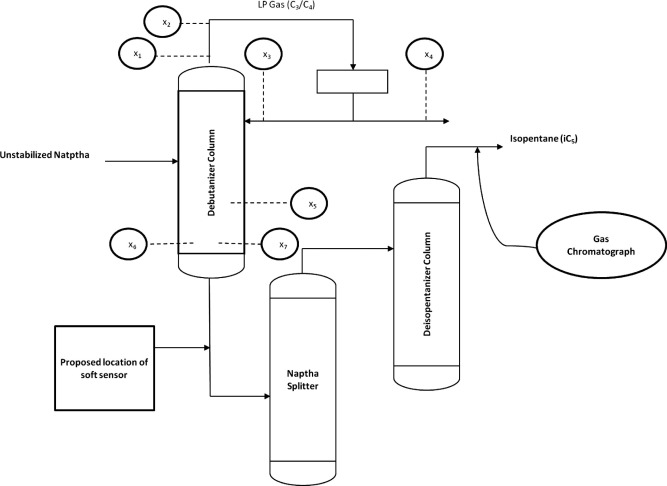Figure 1. Schematic diagram of the debutanizer column.

For improved process performance, the butane (C4) content in the bottom product should be minimized. This requires continuous monitoring of C4 content in the bottom product. A gas chromatograph is used in the process for this purpose. However, the hardware sensor (gas chromatograph) is not installed in the bottom flow line coming from the debutanizer column and instead is located in the overhead of the deisopentanizer column which is located some distance away from the debutanizer column. This introduces a time delay in measurement which is of the order of 30–75 min . Therefore, a soft sensor can be used in the bottom flow of the debutanizer column to overcome the time delay problem of the hardware sensor. The output to be predicted by the soft sensor model is the C4 content present in the debutanizer column bottom product. This output depends on seven process inputs as has been reported in the literature  and . The seven process inputs and the output quality variable to be estimated by the soft sensor are mentioned in Table 2. The location of sensors for the seven process inputs, the gas chromatograph used for C4 content measurement and the proposed soft sensor are shown in Fig. 1.

Table 2. Input–Output Process Variables for the debutanizer column.
Variables Description
Inputs x1 Top temperature
x2 Top pressure
x3 Reflux flow
x4 Flow to next process
x5 6th tray temperature
x6 Bottom temperature
x7 Bottom temperature
Output y Butane (C4) content in the debutanizer column bottom

## 3. Model development

A total of 2394 input–output process data values were available for the debutanizer column. This data set, taken from a petroleum refinery is shared by Fortuna et al. . Interested researchers can access the data from the web resource. The available dataset was equally divided into a training set (used for model development) and a validation set (for model evaluation). Each data subset has 1197 input–output data values. From the total data, the training set was obtained by applying the Kennard–Stone algorithm. The required MATLAB code for implementation of the algorithm was adopted from the freely available TOMCAT toolbox . This training set was subsequently used for development of statistical (multiple linear and principal component) and neural network models.

In multiple linear regression (MLR) model, the output is expressed as a linear combination of the inputs. The MLR model for the debutanizer column has the following form:

 $y={\beta }_{0}+{\beta }_{1}x_{1}+{\beta }_{2}x_{2}+{\beta }_{3}x_{3}+$${\beta }_{4}x_{4}+{\beta }_{5}x_{5}+{\beta }_{6}x_{6}+{\beta }_{7}x_{7}$
(1)

Here, ${\textstyle {\beta }_{0}\ldots {\beta }_{7}}$ are regression coefficients, ${\textstyle x_{1}\ldots x_{7}}$ are process inputs as mentioned in Table 2 and y is the process output i.e. C4 content in the debutanizer column bottom product. The regression coefficients of the above model are determined using the least of squared error criterion as per the equation given below:

 $\beta ={\left(X^{T}X\right)}^{-1}X^{T}Y$
(2)

Here, X is the 1197 × 7 input data matrix, Y is the 1197 × 1 output column vector and β is 7 × 1 column vector consisting of the regression coefficients.

For development of principal component regression (PCR) model, initially principal component analysis (PCA) was conducted on the total input data. The principal components are found by calculating the eigenvectors and eigen values of the data covariance matrix. Subsequently, using cumulative variance criterion principal components or latent variables were selected which are the linear combinations of the actual variables. Least square regression model was developed as mentioned earlier using the latent variables as inputs and the output. The sequence of steps for developing a PCR model from the input–output data set is presented in Fig. 2.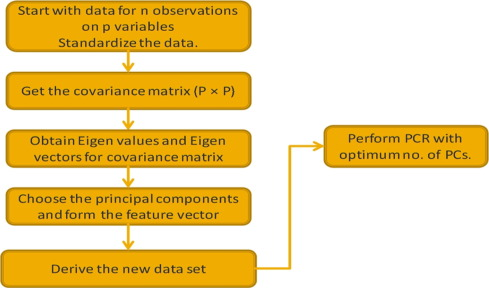Figure 2. Procedure for development of PCR model for any process.

In addition to the MLR and PCR models, back propagation neural network (BPNN) model of the debutanizer column was developed. In a BPNN model, the number of input and output nodes is decided based on process conditions. For the debutanizer column model, the number of input nodes is seven and output node is one. The crucial design step is to optimally determine the number of hidden layer neurons. The activation functions used for the hidden layer and the output layer are, hyperbolic tangent and linear respectively. For deciding optimum number of neurons in hidden layer, the network was initially trained from 3 neurons in hidden layer to 40 neurons in hidden layer using gradient descent training algorithm. The optimum number of neurons was decided as the one which produced lowest error value for the validation data. Subsequently, feed-forward neural networks were created with this optimum number of neurons and trained using three training algorithms. The training algorithms used are as follows: gradient descent, conjugate gradient and Levenberg–Marquardt techniques. The optimum model was the one that produced the lowest error for the validation data i.e. the model with the best generalization capability.

## 4. Results and discussion

The linear regression model developed is as follows:

 $y=0.64+0.422x_{1}-0.402x_{2}-0.134x_{3}+0.238x_{4}-0.481x_{5}-$$0.391x_{6}+0.521x_{7}$
(3)

The number of principal components was determined by analyzing the variance accounted for by the individual principal components. The results of the principal component analysis for the debutanizer column data are reported in Table 3 and the SCREE plot showing the eigenvalue versus principal components is presented in Fig. 3.

Table 3. PCA results of the input data.
Principal components Eigen value Percentage of eigenvalue Cumulative percentage
PC1 2.8723 41.0329 41.0329
PC2 1.4633 20.9039 61.9368
PC3 1.1421 16.3151 78.2519
PC4 0.7441 10.6300 88.8820
PC5 0.5565 7.9496 96.8316
PC6 0.2130 3.0428 99.8744
PC7 0.0088 0.1256 100.0000
Total 7 100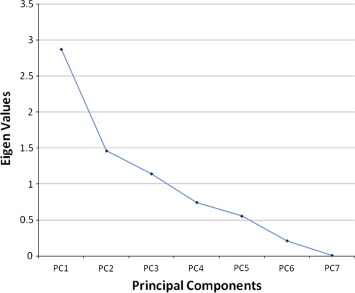Figure 3. SCREE plot for the PCA conducted on the debutanizer column data.

In Table 3, each of the seven principal components is a linear combination of the seven actual input process variables. The usual practice is to retain the number of PCs which have a cumulative variance of more than 70% of the actual total data variance. Therefore, based on the results reported in Table 3 and Fig. 3, five principal components accounting for more than 95% of the total data variance were used for development of PCR model. The principal component regression model obtained has the following form:

 $y=1.32\times {10}^{-5}-0.00059{\mbox{PC}}_{1}-0.03076{\mbox{PC}}_{2}-$$0.02848{\mbox{PC}}_{3}-0.02404{\mbox{PC}}_{4}-0.06074{\mbox{PC}}_{5}$
(4)

As stated earlier, the optimum number of hidden layer neurons in BPNN model was decided by creating models with different number of neurons, training with gradient descent algorithm and simulating the trained networks with the validation data. The results are reported in terms of mean absolute error (MAE) for the training data and validation data in Fig. 4.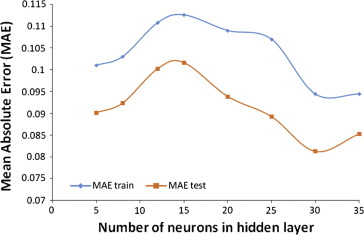Figure 4. MAE for different no. of neurons in hidden layer.

From Fig. 4, it is evident that both testing and training error increases if the number of neurons is increased beyond 30. Therefore optimum number of neurons was decided as 30 with hyperbolic tangent activation function. Keeping this optimum number of neurons fixed two more neural networks were trained using conjugate gradient and Levenberg–Marquardt algorithms. The neural network model was designed using mean absolute error (MAE) as the performance criterion. MAE in this work was determined using the formula given below:

 ${\mbox{MAE}}={\frac {{\sum }_{i=1}^{N}\left|y_{i}-{\overset {\mbox{ˆ}}{y}}_{i}\right|}{N}}$
(5)

Here yi   and ${\textstyle {\overset {\mbox{ˆ}}{y}}_{i}}$ are actual and model predicted values for the ith observation and N is the number of observations.

A model showing better value of one model evaluation parameter may produce worse value of another model evaluation parameter. Therefore, to choose the best model, in addition to MAE, the parameters root mean squared error (RMSE) and correlation coefficient (R) values were computed for all the developed models. The expressions for RMSE and R are presented below:

 ${\mbox{RMSE}}={\sqrt {\frac {{\sum }_{i=1}^{N}{\left(y-{\overset {\mbox{ˆ}}{y}}\right)}^{2}}{N}}}$
(6)
 $R=\left({\frac {\sum (y-{\overline {y}})({\overset {\mbox{ˆ}}{y}}-{\overline {\overset {\mbox{ˆ}}{y}}})}{\sqrt {\sum {\left(y-{\overline {y}}\right)}^{2}\sum {\left({\overset {\mbox{ˆ}}{y}}-{\overline {\overset {\mbox{ˆ}}{y}}}\right)}^{2}}}}\right)$
(7)

The performances of the multiple linear regression (MLR) model, principal component regression (PCR) model and the three neural network models (trained by gradient descent, conjugate gradient and Levenberg–Marquardt algorithms) for training and validation data are reported in Tables 4 and 5 respectively.

Table 4. Debutanizer column model performance for training data.
Model type Statistical model evaluation parameter
Mean absolute error (MAE) Root mean squared error (RMSE) Correlation coefficient (R)
Multiple linear regression (MLR) 0.994 1.007 0.313
Principal component regression (PCR) 0.171 0.24 0.015
Back propagation neural network (BPNN) trained by Gradient-descent 0.094 0.144 0.537
Levenberg–Marquardt 0.046 0.064 0.925

Table 5. Debutanizer column model performance for validation data.
Model type Statistical model evaluation parameter
Mean absolute error (MAE) Root mean squared error (RMSE) Correlation coefficient (R)
Models reported in this work Multiple linear regression (MLR) 0.989 0.999 0.395
Principal component regression (PCR) 0.105 0.1511 0.148
Back propagation neural network (BPNN) trained by Gradient-descent 0.081 0.125 0.553
Levenberg–Marquardt 0.055 0.076 0.856
Models reported by Ge and Song  LSSVR Not reported 0.1418 0.9132
SVR Not reported 0.145 0.6897
PLS Not reported 0.165 0.4035
Model reported by Ge et al.  Non-linear semi supervised PCR Not reported 0.1499 Not reported

MAE values are reported because it has been mentioned in the literature that MAE is a better model evaluation parameter as compared to other statistical parameters . Comparison of the MAE values of different models shows that the BPNN model trained using Levenberg–Marquardt algorithm, clearly outperforms all other models. The accuracy of the BPNN model is also quite satisfactory. The average error produced by the model is 4.6% for the training data and 5.5% for the validation data.

The purpose of reporting the other two statistical parameters is for the sake of comparison with values reported in the literature. Usually, comparing the performances of different data driven models is difficult, because the data used are different for different models reported in the literature. However, in this research, use of the same benchmark data set for the debutanizer column soft sensor development by various researchers offers scope for comparison of the different model performances. In the last two rows of Table 5 the different statistical values reported by Ge and Song  and Ge et al.  are reproduced. The RMSE values mentioned for the model reported in  are approximate values since the values were available only in the form of a chart. It may be noted that the R value of the LSSVR model is slightly better than the R value of the best model reported in this work. However the present BPNN model is superior to the LSSVR and PCR models reported earlier in terms of the RMSE value.

Finally in Figure 5 and Figure 6 the prediction results of the present BPNN model are reported for the training and validation data set respectively.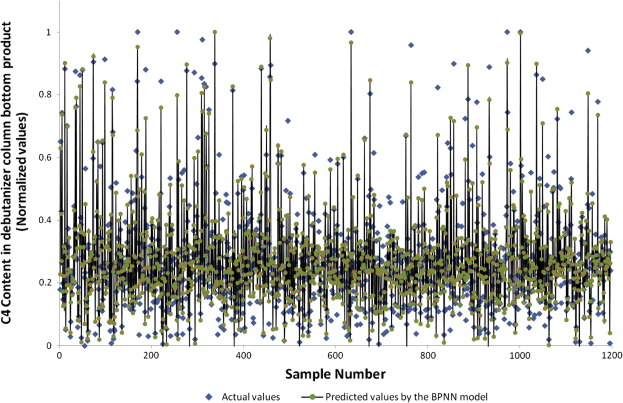Figure 5. Prediction of C4 content in the debutanizer bottom product (training data).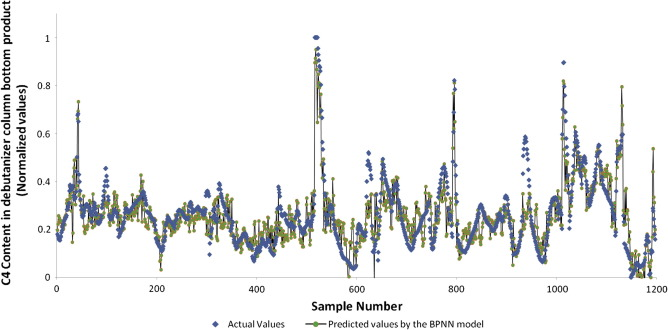Figure 6. Prediction of C4 content in the debutanizer bottom product (validation data).

The exact neural network model developed in this work is represented in Fig. 7. The model receives information of seven process variables as inputs and produces the estimated value of C4 content in the debutanizer column bottom product.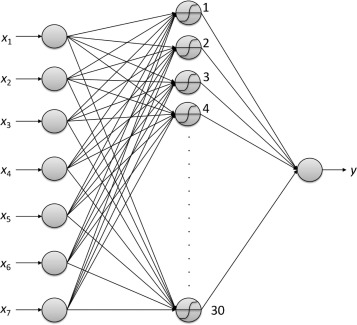Figure 7. Feed forward neural network model of the debutanizer column.

For the sake of verification of the reported results and further research, the architecture of the present optimized neural network model, weight values for the hidden and outer layers and the input–output data used for model development and validation are supplied as supplementary materials.

The architecture of the neural network model is as follows: seven input nodes, thirty hidden layer nodes and 1 output node. Activation functions used are hyperbolic sigmoidal in hidden layer neurons and linear in output neuron. The weights associated with the neural network structure are given below:

• Input to hidden layer: 7 × 30 weight matrix.
• Bias values to hidden layer neurons: 30 × 1 weight vector.
• Hidden layer to output layer: 1 × 30 weight vector.
• Bias value to output layer neuron: 1 × 1 (single scaler value).

All weight values of the trained neural network model are provided as supplementary materials. Interested readers can download the process data supplied by Fortuna et al.  from the website, create the feed forward neural network model with the structure and weight values supplied in this article and verify the results. They are also encouraged to bring further improvements in the modeling results.

## 5. Conclusion

For optimum process performance the content of butane in the debutanizer column bottom product should be limited to a minimum. This control of product quality requires online monitoring of the product composition. However, the hardware sensor (gas chromatograph) used for composition monitoring is located some distance far from the column and hence introduces significant time delay in the monitored value. Therefore, a soft sensor installed in the product outlet line can be effectively used for continuous monitoring and control of product quality. A back propagation neural network based soft sensor trained with Levenberg–Marquardt algorithm is reported in this article. Simulation study shows that the reported soft sensor performs better than the multiple linear regression and principal component regression models reported in this work and the earlier reported models of least square support vector regression and non-linear semi supervised principal component regression models reported earlier in the literature.

## Appendix A. Supplementary material

Supplementary data 1.

### Document informationPublished on 12/04/17

Licence: Other

### Document Score0

Views 15
Recommendations 0